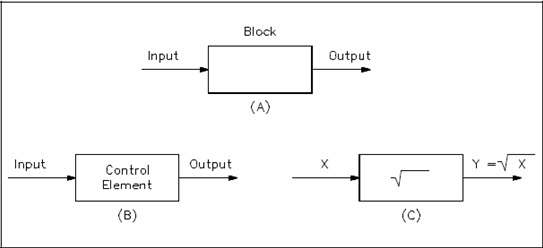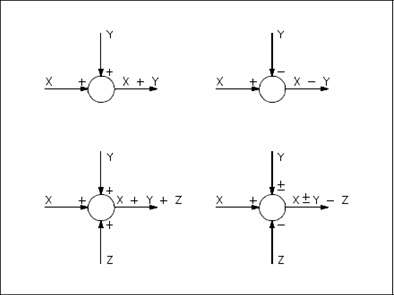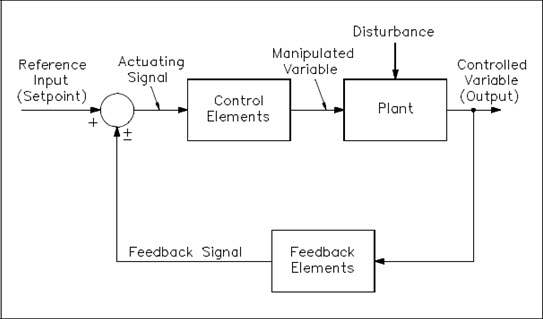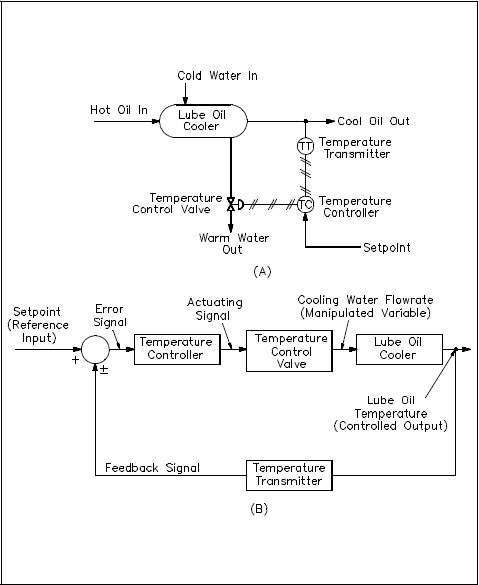Process Control Technicians are in high demand in industry.  As automation continues to advance our capabilities, it also increases the difficulty of maintaining the system.  Understanding the complex systems in automation begins with the basics, such as print reading.

block diagram is a pictorial representation of the cause and effect relationship between the input and output of a physical system. A block diagram provides a means to easily identify the functional relationships among the various components of a control system.

The simplest form of a block diagram is the block and arrows diagram. It consists of a single block with one input and one output (Figure 1A). The block normally contains the name of the element (Figure 1B) or the symbol of a mathematical operation (Figure 1C) to be performed on the input to obtain the desired output. Arrows identify the direction of information or signal flow.Figure 1 Block and Arrows

Although blocks are used to identify many types of mathematical operations, operations of addition and subtraction are represented by a circle, called a summing point. As shown in Figure 2, a summing point may have one or several inputs. Each input has its own appropriate plus or minus sign. A summing point has only one output and is equal to the algebraic sum of the inputs.Figure 2 Summing Points

takeoff point is used to allow a signal to be used by more than one block or summing point (Figure 3).Figure 3 Takeoff Point

Feedback Control System Block Diagram

Figure 4 shows basic elements of a feedback control system as represented by a block diagram. The functional relationships between these elements are easily seen. An important factor to remember is that the block diagram represents flowpaths of control signals, but does not represent flow of energy through the system or process.Figure 4 Feedback Control System Block Diagram

Below are several terms associated with the closed-loop block diagram.

• The plant is the system or process through which a particular quantity or condition is controlled. This is also called the controlled system.
• The control elements are components needed to generate the appropriate control signal applied to the plant. These elements are also called the “controller.”
• The feedback elements are components needed to identify the functional relationship between the feedback signal and the controlled output.
• The reference point is an external signal applied to the summing point of the control system to cause the plant to produce a specified action. This signal represents the desired value of a controlled variable and is also called the “setpoint.”
• The controlled output is the quantity or condition of the plant which is controlled. This signal represents the controlled variable.
• The feedback signal is a function of the output signal. It is sent to the summing point and algebraically added to the reference input signal to obtain the actuating signal.
• The actuating signal represents the control action of the control loop and is equal to the algebraic sum of the reference input signal and feedback signal. This is also called the “error signal.”
• The manipulated variable is the variable of the process acted upon to maintain the plant output (controlled variable) at the desired value.
• The disturbance is an undesirable input signal that upsets the value of the controlled output of the plant.

Figure 5 shows a typical application of a block diagram to identify the operation of a temperature control system for lubricating oil. Figure 5A shows a schematic diagram of the lube oil cooler and its associated temperature control system.Figure 5 Lube Oil Cooler Temperature Control System and Equivalent Block Diagram